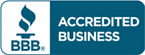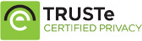# Solution manual for Business Statistics 9th edition David F. Groebner

\$30.00

Business Ethics Ethical Decision Making & Cases, 9th Edition by O. C. Ferrell Solutions manual, answers key to end of chapter questions

You want Test bank?## Description

Solution manual for Business Statistics 9th edition David F. Groebner

Table of content

1. The Where, Why, and How of Data Collection
2. Graphs, Charts, and Tables–Describing Your Data
3. Describing Data Using Numerical Measures
4. Introduction to Probability
5. Discrete Probability Distributions
6. Introduction to Continuous Probability Distributions
7. Introduction to Sampling Distributions
8. Estimating Single Population Parameters
9. Introduction to Hypothesis Testing
10. Estimation and Hypothesis Testing for Two Population Parameters
11. Hypothesis Tests and Estimation for Population Variances
12. Analysis of Variance
13. Goodness-of-Fit Tests and Contingency Analysis
14. Introduction to Linear Regression and Correlation Analysis
15. Multiple Regression Analysis and Model Building
16. Analyzing and Forecasting Time-Series Data
17. Introduction to Nonparametric Statistics
18. Introduction to Quality and Statistical Process Control

## Reviews

There are no reviews yet.